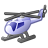Choose Language Hide Translation Bar
Highlighted

## Reference Lines that Update With Change in Local Data Filter

Hi,

I have time series data where chunks of time are broken up into different "cycles", and I'm trying to find a way to create references lines marking the beginning of each cycle. I want these reference lines to update when I change the local data filter across trials (because the cycles start at different times for the different trials).

At first, I was using a local data filter, detecting changes in the local data filter using Make Row State Handler. However I couldn't figure out how to get the necessary information from the local data filter (i.e. what trial was being filtered—although I might have gotten close using Get Where Clause on the local data filter) in and so was unable to know the trial that was being filtered and have this dictate which cycle times to use for creating the reference lines. (however if you think this is a better approach, let me know).

So, instead of having the user interact with the local data filter and trying to read the filter to get the trials being filtered, I decided I'd provide a list of the trials to the user, then add a filter based on what trial the user selected, while at the same time using the selection to dictate which cycle times to use to create the reference lines.

However, I'm unable to to add reference lines that change when the user selects a different trial number.

``````//Reference Lines that Update With Change in Local Data Filter
// Example Data
dt = New Table( "Example",
New Column( "Trial",
character,
"Ordinal",
Set Values( {"A","A","A","A","A","A","A","A","A","A","A","A","A","A","B","B","B","B","B","B","B","B","B","B","B","B","B","B"} )
),
New Column( "Cycle",
Character,
"Ordinal",
Set Values( {"Rinse","Rinse","Rinse","Wash","Wash","Wash","Wash","FinalRinse","FinalRinse","FinalRinse","FinalRinse","Dry","Dry","Dry","Rinse","Rinse","Rinse","Wash","Wash","Wash","Wash","FinalRinse","FinalRinse","FinalRinse","FinalRinse","Dry","Dry","Dry
"} )
),
New Column( "%Clean",
Numeric,
"Continuous",
Set Values( {10,20,25,50,60,70,80,85,90,95,99,99,99,99,10,25,30,60,75,85,90,95,99,99.9,99.99,99.99,99.99,99.99} )
),
New Column( "Time",
Numeric,
"Continuous",
Set Values( {1,2,3,4,5,6,7,8,9,10,11,12,13,14,2,4,6,8,10,12,14,16,18,20,22,24,26,28} )
),
);

dt = current data table();

//Function to pull cycle start times (where reference lines will be drawn)
genRefLineValue = function({trialNum = "A", cycleNum = "Rinse"},{myrows,myvalues,min_value},
myrows = dt << get rows where(and(:Trial == trialNum,:Cycle == cycleNum));
myvalues = :Time[myrows];
min_value = min(myvalues);
Return(min_value);
);

//Function to pull unique cycles (I want the start time for EACH of the cycles)
findUniqueCycles = function({trialNum},{myrows,cycles,uniqueCycles},
myrows = dt << get rows where(:Trial == trialNum);
cycles = :Cycle[myrows];
uniqueCycles = associative array(cycles) << get keys;
return(uniqueCycles);
);

//Get unique trials (A, B, etc.)
summarize(unique_values=by(:Trial));

//Create window with radio buttons and graph
New Window( "Example",
HListBox(
unique_values, <<set(1)

),
Text Box( "" )
),
biv = dt << Bivariate( Y( :name("%Clean") ), X( :name("Time") )),//Adding graph
biv << Local Data Filter(invisible, //Adding local data filter that will change according to what user selects with radio buttons
columns( :Trial ),
Where( :Trial == "A" ),
)),

),

);

rb << Set Function(
Function( {this},{myChoice},
myChoice = Char( this << get selected );
(this << sib) << Set Text( myChoice );
//print(myChoice);
biv << remove local data filter;
biv << Local Data Filter(invisible, //updating (by removing then re-adding) the local data filter according to user's selection
columns( :Trial ),
Where( :Trial == myChoice ),
));
)
);

report(biv)[framebox(1)] << Frame Size( 1000, 400 );

//I'm able to add reference lines that don't change - but I want it to change based on the user selection of the radio buttons
For(i=1,i<= (n items (findUniqueCycles("A"))),i++,
cycleName = findUniqueCycles("A")[i];
cycleStart = genRefLineValue("A",findUniqueCycles("A")[i]);
report(biv)[axisbox(2)] << add ref line(cycleStart,"solid",,cycleName ||"(t="||char(cycleStart)||" min)",1.5);

);``````

At the bottom is where I add the reference lines. If you run the script and select between Trial A and B, you can see that while the filter's working and the data changes accordingly, the reference lines don't change (it might look like they change, but if you observe the actual values, they aren't changing). I've tried using myChoice in place of "A" so that the arguments in the loop that is creating the reference lines should update when the user selects Trial B:

``````For(i=1,i<= (n items (findUniqueCycles(myChoice))),i++,
cycleName = findUniqueCycles(myChoice)[i];
cycleStart = genRefLineValue(myChoice,findUniqueCycles(myChoice)[i]);
report(biv)[axisbox(2)] << add ref line(cycleStart,"solid",,cycleName ||"(t="||char(cycleStart)||" min)",1.5);

);``````

But JMP complains by saying:

`Name Unresolved: myChoice{3} in access or evaluation of 'myChoice' , myChoice/*###*/`

How do I make reference lines that update when the user changes their selection among trials?

Highlightedpeng_liu
Staff

## Re: Reference Lines that Update With Change in Local Data Filter

I think you are almost there.

Try replace the later portion of your codes by this

``````
rb << Set Function(
Function( {this},{myChoice},
myChoice = Char( this << get selected );
(this << sib) << Set Text( myChoice );
//print(myChoice);
biv << remove local data filter;
biv << Local Data Filter(invisible, //updating (by removing then re-adding) the local data filter according to user's selection
columns( :Trial ),
Where( :Trial == myChoice ),
));

if (myChoice == "A",
For(i=1,i<= (n items (findUniqueCycles("B"))),i++,
cycleStart = genRefLineValue("B",findUniqueCycles("B")[i]);
report(biv)[axisbox(2)] << remove ref line(cycleStart);

)
);

if (myChoice == "B",
For(i=1,i<= (n items (findUniqueCycles("A"))),i++,
cycleStart = genRefLineValue("A",findUniqueCycles("A")[i]);
report(biv)[axisbox(2)] << remove ref line(cycleStart);
)
);

For(i=1,i<= (n items (findUniqueCycles(myChoice))),i++,
cycleName = findUniqueCycles(myChoice)[i];
cycleStart = genRefLineValue(myChoice,findUniqueCycles(myChoice)[i]);
report(biv)[axisbox(2)] << add ref line(cycleStart,"solid",,cycleName ||"(t="||char(cycleStart)||" min)",1.5);

);
)
);

report(biv)[framebox(1)] << Frame Size( 1000, 400 );

//you need this to initialize somehow
For(i=1,i<= (n items (findUniqueCycles("A"))),i++,
cycleName = findUniqueCycles("A")[i];
cycleStart = genRefLineValue("A",findUniqueCycles("A")[i]);
report(biv)[axisbox(2)] << add ref line(cycleStart,"solid",,cycleName ||"(t="||char(cycleStart)||" min)",1.5);

);

``````

In addition, the error means myChoice cannot be found or undefined, because you used it outside of where it is available, which is inside of a callback.

Article Labels